# How to add and subtract dates, days, weeks, months and years in Excel

In this tutorial, you will find a variety of useful formulas to add and subtract dates in Excel, such as subtracting two dates, adding days, weeks, months and years to a date, and more.

If you have been following our tutorials to working with dates in Excel, you already know an array of formulas to calculate different time units such as days, weeks, months and years.

When analyzing the date information in your worksheets, you are likely to perform some arithmetic operations with those dates too. This tutorial explains a few formulas for adding and subtracting dates in Excel that you may find useful.

## How to subtract dates in Excel

Supposing you have two dates in cells A2 and B2, and now you want to subtract one date from the other to know how many days are between these dates. As is often the case in Excel, the same result can be achieved in several ways.

#### Example 1. Subtract one date from the other directly

As you probably know, Microsoft Excel stores each date as a unique serial numbers beginning with 1 that represents January 1, 1900. So, you are actually subtracting two numbers, and an ordinary arithmetic operation works without a hitch:

`=B2-A2`

#### Example 2. Subtract dates using Excel DATEDIF function

If the above formula looks too plain, you can achieve the same result in a guru-like way by using Excel's DATEDIF function:

`=DATEDIF(A2, B2, "d")`

The following screenshot demonstrates that both calculations return identical results, except for row 4 where the DATEDIF function returns the #NUM error. Let's figure out why that happens.

When you subtract a more recent date (6-May-2015) from an earlier date (1-May-2015), the subtraction operation returns a negative number (-5) exactly as it should. The syntax of the Excel DATEDIF function, however, does not allow the start date to be greater than the end date and therefore it returns an error.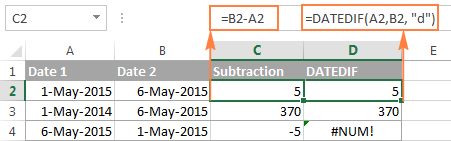#### Example 3. Subtract a date from the current date

To subtract a date from today's date, you can employ either of the above formulas. Just use the TODAY() function instead of date 1:

`=TODAY()-A2`

or

`=DATEDIF(A2,TODAY(), "d")`

Like in the previous example, both formulas work fine when today's date is greater than the date you are subtracting from it, otherwise DATEDIF fails: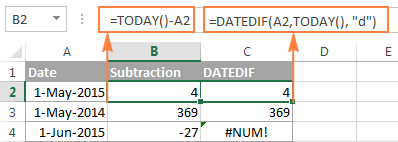#### Example 4. Subtracting dates with Excel DATE function

If you prefer to supply the dates directly in the formula, then enter each date using the DATE(year, month, day) function and then subtract one date from the other.

For instance, the following formula subtracts 15-May-2015 from 20-May-2015 and returns the difference of 5 days:

`=DATE(2015, 5, 20) - DATE(2015, 5, 15)`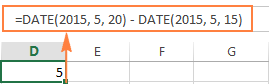Wrapping up, when it comes to subtracting dates in Excel and you want to find out how many days are between two dates, it makes sense to go with the easiest and most obvious option - simply subtract one date directly from another.

If you are looking to count the number of months or years between two dates, then the DATEDIF function is the only possible solution and you will find a few formula examples in the next article that will cover this function in full details.

Now that you know how to subtract two dates, let's see how you can add or subtract days, months, or years to a given date. There are a number of Excel functions suitable for this purpose, and which one you use depends on which unit you want to add or subtract.

## How to subtract or add days to date in Excel

If you have a date in some cell or a list of dates in a column, you can add or subtract a certain number of days to those dates using a corresponding arithmetic operation.

#### Example 1. Adding days to a date in Excel

The general formula to add a specified number of days to a date in as follows:

Date + N days

The date can be entered in several ways:

• As a cell reference, e.g. `=A2 + 10`
• Using the DATE(year, month, day) function, e.g. `=DATE(2015, 5, 6) + 10`
• As a result of another function. For example, to add a given number of days to the current date, use the TODAY() function: `=TODAY()+10`

The following screenshot demonstrates the above formulas in action. The current date at the moment of writing was 6 May, 2015: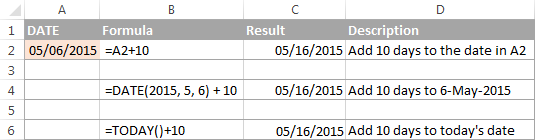Note. The result of the above formulas is a serial number representing the date. To get it displayed as a date, select the cell(s) and press Ctrl+1 to open the Format Cells dialog. On the Number tab, select Date in the Category list, and then choose the date format you want. For the detailed steps, please see How to change date format in Excel.

#### Example 2. Subtracting days from a date in Excel

To subtract a given number of days from a certain date, you perform a usual arithmetic operation again. The only difference from the previous example is that you type the minus sign instead of plus :)

Date - N days

Here are a few formula examples:

• `=A2-10`
• `=DATE(2015, 5, 6)-10`
• `=TODAY()-10`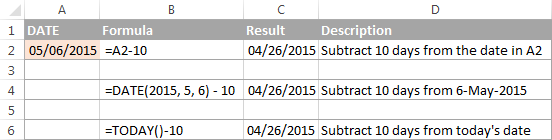## How to add or subtract weeks to date

In case you want to add or subtract whole weeks to a certain date, you can use the same formulas as for adding / subtracting days, and simply multiply the number of weeks by 7:

Adding weeks to a date in Excel:

cell+ N weeks * 7

For example, you add 3 weeks to the date in A2, use the following formula: `=A2+3*7`.

Subtracting weeks from date in Excel:

cell - N weeks * 7

To subtract 2 weeks from today's date, you write `=TODAY()-2*7`.

## How to add / subtract months to date in Excel

If you want to add or subtract a certain number of whole months to a date, you can employ either the DATE or EDATE function, as demonstrated below.

#### Example 1. Add months to a date with Excel DATE function

Taking a list of dates in column A for example, type the number of dates you want to add (positive number) or subtract (negative number) in some cell, say C2.

Enter the following formula in cell B2 and then drag the corner of the cell all the way down to copy the formula to other cells:

`=DATE(YEAR(A2), MONTH(A2) + \$C\$2, DAY(A2))`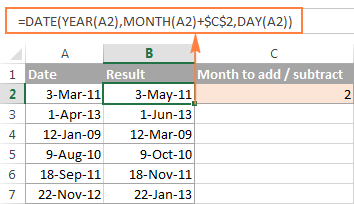Now, let's see what the function is actually doing. The logic behind the formula is obvious and straightforward. The DATE(year, month, day) function takes the following arguments:

• the year of the date in cell A2;
• the month of the date in A2 + the number of months you specified in cell C2, and
• the day of the date in A2.

Yep, it's that simple :) If you type a negative number in C2, the formula will subtract months instead of adding them: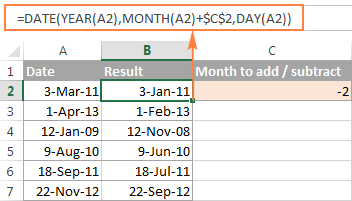Naturally, nothing prevents you from typing the minus sign directly in the formula to subtract months from a date:

`=DATE(YEAR(A2), MONTH(A2) - \$C\$2, DAY(A2))`

And of course, you can type the number of month to add or subtract in the formula instead of referring to a cell:

`=DATE(YEAR(date), MONTH(date) + N months, DAY(date))`

The real formulas could look similar to these:

• Add months to date: `=DATE(YEAR(A2), MONTH(A2) + 2, DAY(A2))`
• Subtract months from date: `=DATE(YEAR(A2), MONTH(A2) - 2, DAY(A2))`

#### Example 2. Add or subtract months to a date with Excel EDATE

Microsoft Excel provides a special function that returns a date that is a specified number of months before or after the start date - the EDATE function. It is available in modern versions of Excel 2007, 2010, 2013 and upcoming Excel 2016.

In your `EDATE(start_date, months)` formulas, you supply the following 2 arguments:

• Start_date - the start date from which to count the number of months.
• Months - the number of months to add (a positive value) or subtract (a negative value).

The following formula used on our column of dates yields exactly the same results as the DATE function in the previous example: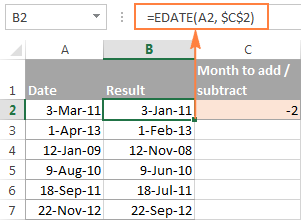When using the EDATE function, you can also specify the start date and the number of month to add / subtract directly in the formula. Dates should be entered by using the DATE function or as results of other formulas. For example:

• To add months in Excel:

`=EDATE(DATE(2015,5,7), 10)`

The formula adds 10 months to 7-May-2015.

• To subtract months in Excel:

`=EDATE(TODAY(), -10)`

The formula subtracts 10 months from today's date.

Note. The Excel EDATE function returns a serial number representing the date. To force Excel to display it as a date, you should apply the Date format to the cells with your EDATE formulas. Please see Changing the date format in Excel for the detailed steps.

## How to subtract or add years to date in Excel

Adding years to a date in Excel is done similarly to adding months. You use the DATE(year, month, day) function again, but this time you specify how many years you want to add:

DATE(YEAR(date) + N years, MONTH(date), DAY(date))

In your Excel worksheet, the formulas may look as follows:

• To add years to a date in Excel:

`=DATE(YEAR(A2) + 5, MONTH(A2), DAY(A2))`

The formula adds 5 years to the date in cell A2.

• To subtract years from a date in Excel:

`=DATE(YEAR(A2) - 5, MONTH(A2), DAY(A2))`

The formula subtracts 5 years from the date in cell A2.

If you type the number of year to add (positive number) or subtract (negative number) in some cell and then refer to that cell in the DATE function, you will get a universal formula: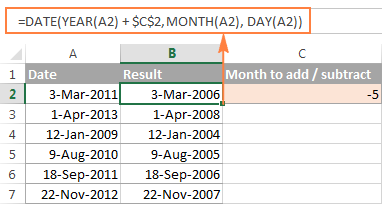## Add / subtract days, months and years to date

If you carefully observed the two previous examples, I think you have already guessed how to add or subtract a combination of years, months and days to a date in a single formula. Yep, using the good old DATE function :)

DATE(YEAR(date) + X years, MONTH(date) + Y months, DAY(date) + Z days)

To subtract years, months, days:

DATE(YEAR(date) - X years, MONTH(date) - Y months, DAY(date) - Z days)

For example, the following formula adds 2 years, 3 months and subtracts 15 days from a date in cell A2:

`=DATE(YEAR(A2) + 2, MONTH(A2) + 3, DAY(A2) - 15)`

Applied to our column of dates, the formula takes the following shape:

`=DATE(YEAR(A2) + \$C\$2, MONTH(A2) + \$D\$2, DAY(A2) + \$E\$2)`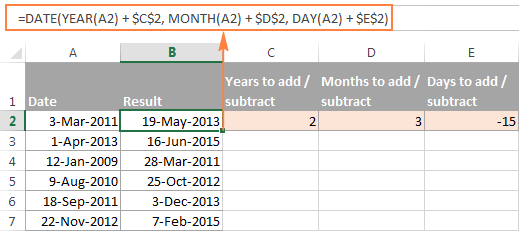## How to add and subtract times in Excel

In Microsoft Excel, you can add or subtract times using the TIME function. It lets you operate on time unites (hours, minutes and seconds) exactly in the same way as you handle years, months and days with the DATE function.

cell + TIME(hours, minutes, seconds)

To subtract time in Excel:

cell - TIME(hours, minutes, seconds)

Where A2 contains the time value you want to change.

For example, to add 2 hours, 30 minutes and 15 seconds to the time in cell A2, you can use the following formula:

`=A2 + TIME(2, 30, 15)`

If you want to add and subtract time unites within one formula, just add the minus sign to the corresponding values:

`=A2 + TIME(2, 30, -15)`

The above formula adds 2 hours and 30 minutes to the time in cell A2 and subtracts 15 seconds.

Alternatively, you can enter the time unites you want to changes in some cells, and refer to those cells in your formula:

`=A2 + TIME(\$C\$2, \$D\$2, \$E\$2)`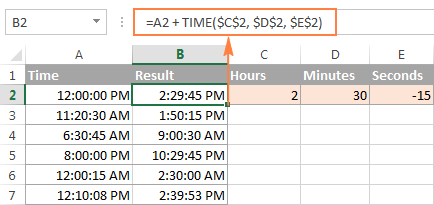If the original cells contain both date and time, the above formula works perfectly too: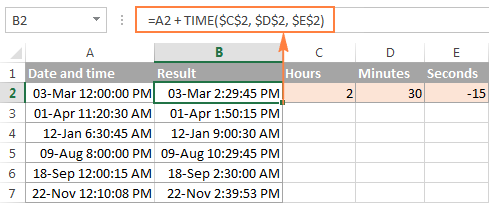## Date & Time Formula Wizard - quick way to add and subtract dates in Excel

Now that you know a bunch of different formulas to calculate dates in Excel, wouldn't you want to have just one that can do all this? Of course, such a formula can never exist. However, there exists the Date & Time Wizard that can build any formula for you on the fly, provided that you have our Ultimate Suite installed in your Excel. Here's how:

1. Select the cell in which you want to insert the formula.
2. Head to the Ablebits Tools tab, and click the Date & Time Wizard button:3. The Date & Time Wizard dialog window shows up. Depending on whether you want to add or subtract dates, switch to the corresponding tab, supply data for the formula arguments, and click the Insert Formula button.

As an example, let's add a few months to the date in cell A2. For this, you go to the Add tab, type A2 in the Enter a date box (or click in the box and select the cell on the sheet), and type the number of months to add in the Month box.

The wizard makes a formula and shows its preview in the cell. It also shows the calculated date under Formula result: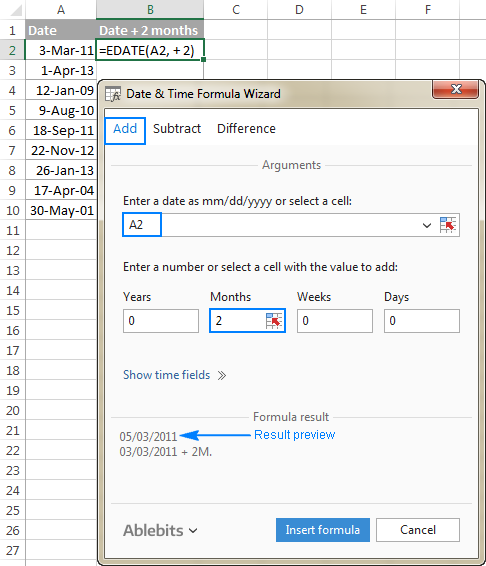If you are satisfied with the result, click the Insert formula button. Once the formula is added, you can copy it to as many cells as necessary: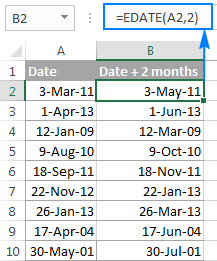That was quite a simple formula, wasn't it? Let's give the wizard something more challenging to work on. For example, let us subtract some years, months, weeks and days from the date in A2. To have it done, switch to the Subtract tab and type the numbers in the corresponding boxes. Or you can enter the units in separate cells and supply references to those cells, as shown in the screenshot below: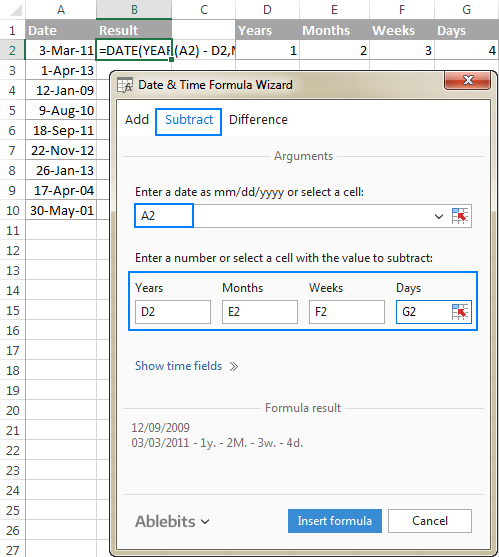Clicking the Insert formula button inputs the following formula in A2:

`=DATE(YEAR(A2)-D2,MONTH(A2)-E2,DAY(A2)-G2-F2*7)`

If you plan to copy the formula to other cells, you have to change all cell references except A2 to absolute references so that the formula copies correctly (by default, the wizard always uses relative references). To fix the reference, you simply type the \$ sign before the row and column coordinates, like this:

`=DATE(YEAR(A2)-\$D\$2,MONTH(A2)-\$E\$2,DAY(A2)-\$G\$2-\$F\$2*7)`

And get the following results: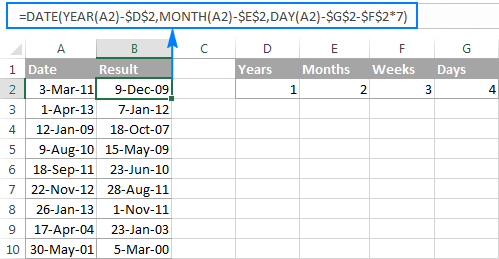Additionally, you can click the Show time fields link and add or subtract date and time units with one formula.

If you wish to play with the Date & Time Formula Wizard in your own worksheets, you are welcome to download the 14-day trial version of the Ultimate Suite.

This is how you add and subtract dates in Excel. I am hopeful you have learned a couple of useful functions today. I thank you for reading and hope to see you on our blog next week.

## You may also be interested in

Category: Excel Tips

### 202 responses to "How to add and subtract dates, days, weeks, months and years in Excel"

1.Joanne says:

I have an Excel Spreadsheet that I am using to track success completing multiple tasks by companies. I have built a spreadsheet with a "Date Due" and a "Date Completed" column for each task. I already know the due dates and have populated the same. The dates items are completed are entered when done. I then calculate the difference between the Date Due and the Date Completed. I have built the simple formula of =Sum(D4-E4). I built the spreadsheet and copied the formula to all columns/cells that will eventually be populated. Here is my question, how do I get the cells that include the formula to return a 0 or nil value if the E4 value in the formula above is not populated? This means my work is still in progress and I don't have a "Date Completed" value. I am assuming an IF statement will do it, but I would like some guidance/recommendation . . .

Thanks,

•Gennady Terekhov (Ablebits.com Team) says:

Hello, Joanne,

=IF(ISBLANK(E4),"0",E4)

2.Sangram says:

Do you have any formula to increment year of date by some no. like EDATE for month ?

3.T.Dhanasekar says:

When I tried formula =datedif(Cell2,cell3,"d") it displays result as #value!

Kindly help me in sorting out this issue I want to calculate number of days between eg.18/11/2017 to 1/1/2018

4.kds14589 says:

I have a formula that needs to be performed on dates every month that are larger than a date I have listed by a number, such as every 8th (8)or 22nd (22), and this is preformed again for each new month. My problem is having excel distinguish between a today() format and my number. Any recommendations?

•Gennady Terekhov (Ablebits.com Team) says:

Hello,
For me to understand the problem better, please send me a small sample workbook with your source data and the result you expect to get to support@ablebits.com. Please don't worry if you have confidential information there, we never disclose the data we get from our customers and delete it as soon as the problem is resolved.

5.Joseph says:

What formula should I use for the given scenario:

Date on Cell 2 should be either 16th of the next month or 1st of the 2nd next month.

1st to 15th of the month = 16th of the next month
16th to 31st of the month = 1st of the 2nd next month

For example, date in cell 1 is january 10, 2017, the date on cell 2 should be feb 16, 2017. If the date on cell 1 is january 26, 2017, the date on cell 2 should be march 1, 2017.

Thank you.

6.steve newman says:

Is there an easy way to calculate the number of month's, weeks, hours & minutes from a date to a date? This will cover leap years & any other variances I haven't already though of?

TIA

7.ola says:

Kindly help me in sorting out this issue I want to calculate number of days between eg.29/11/2017 to 09/11/2017

•Leigh Jackson says:

Just do latest date - earliest date
e.g. Cell A1 contains 29/11/2017, Cell B2 contains 09/11/2017
Do =A1-B2 the result will be the number of days (ensure the result is in 'General' format)

8.aad says:

very helpful, didnt find it in excel help, thanks alot

9.Robert says:

Hello. I have created a spreadsheet to record calculations for when to reorder tablet medication. The spreadsheet opens with Todays date but doesnt automatically look at the last stock count date and reflect actually how many tablets are left in the box......for me to reorder.

I would like the formula for todays date cell to delete the stock count date cell and then delete the quantity of tablets number cell already recorded please.

Thanks

10.Karen says:

Hi, I'm looking for a formula to calculate when a payment is due when it's 30 days after month end.
Eg.. Invoice date 15th May,the due date would be 30 days after month end..
So I trust the actual date would be 30th June.. Is there a formula for this.?

•Doug says:

Karen:
The formula you're looking for is:
=EOMONTH(A22,1)
Where 5/15/18 is in A22 and is formatted as Date as is the cell containing the formula.

11.Frank Sikazwe says:

I have the following in cells A1 (start date and time) and B1 (finish date and time)
A1: 29/12/2017 11:44
B1: 02/01/2018 08:00

What formula can I use to get the total time from A1 to B1 in HH:mm?

•Frank Sikazwe says:

I found this to work though giving decimal values
=DAYS(B1,A1)*24+HOUR(B1-A1)+MINUTE(B1-A1)/60

12.Shy says:

I need to know the no. of days between dates (with 1 days equivalent to 8 hours) and hours between time and combining these 2 to give me the total no. of days and hours in one cell.

Given: 7/18/2018 - 7/20/2018 = no. of days
8:00:00 - 12:00:00 = no. of hours
Result should be in no. of days and hours (in one cell)

13.Shy says:

I need to know the no. of days between dates (with 1 days equivalent to 8 hours) and hours between time and combining these 2 to give me the total no. of days and hours in one cell.

Given: 7/18/2018 - 7/20/2018 = no. of days
8:00:00 - 12:00:00 = no. of hours
Result should be in no. of days and hours (in one cell)

14.Gold Mobolaji says:

hi there, how can i make certain words fall automatically under a category.
for example i have spoon, fork, knife, plate, bowl, saucer, i want the first 3 to always specify cutlery in their next column and the last 3 to always specify dishes in their next column any time they are typed the next column should automatically fill the category they belong.

15.Joe From in Bryant says:

Hi there,
I am setting up my class assignment schedule in excel but I have difficulty to setup a formula that will reduce the date till the deadline is reached. Please help me on this.

16.Joe Bryant says:

Hi there,
I am setting up my class assignment schedule in excel but I have difficulty to setup a formula that will reduce the date till the deadline is reached. Please help me on this.

17.Russell says:

I am looking to have one column have the name of the months, i.e., "January." What I would like in column two is to have column one minus 3 months. So in the example January in column one, column two would show October (January minus three months).

18.janisar says:

I want to subtract and calculate difference hours these two date
Oct 14, 2018, 2:00 PM - Nov 1, 2018, 2:20 PM

19.Yashwant says:

Thanks a lot! I was searching a formula to add months in a particular date. I could find the right formula here.

20.Andy R says:

Is it possible to change the colour of a box when a date is reached ?
This is to keep track of machine servicing, I want to enter a date when the service was done and the box to show green until a year has elapsed when the box would change to red.

21.Wan says:

I would like to calculate number of days from start date to end date, covering all days.
for example; Travelling dates from 1 Mar to 5 Mar = 6 days
what formula does suit to calculate?

Thank you

22.Nitin Chandra says:

I want to subtract two dates like this- Tue Oct 23 21:44:03 BST 2018
How can I do it please?

23.Wim says:

I need to calculate the total deal cost for deals with a start date and end date and an annual recurring rate. Leap years need to be taken into account. Deals are usually between 12 months and 48 months. I would like to see a result like 3.26 year where all years are calculated as if they are being 365 days. Hope you can help.

24.Mohaimenul says:

how I convert number 20 to 1 year 8 months
also 38 to 3 year 2 months
hope you can help..

25.Daisy says:

Hi all,

I have a spreadsheet that has an issue date, importance rating and then a review date. What i'd like to happen is that if the importance rating is high then the review date needs to be the issue date plus 1 year, and then so on with all the other options but the one i have used isn't working and adding YEAR doesn't seem to be working either....

Here is what i have:
=IF([@Importance]="High",[@[Issue Date]]+1,IF([@Importance]="Medium",[@[Issue Date]]+3,IF([@Importance]="Low",[@[Issue Date]]+5,"N/A")))

Any help would be great!! :)

26.kiranMV says:

HI,
I would like to get the difference in the dates "4/13/2019" and "2/25/2019" so used function "=DAYS("4/13/2019","2/25/2019")", but getting the result like this .."2/16/1900", i was expecting 47, but no matter which function(Tried with today(), simply subtraction) i am using, i am seeing the same result.. can some explain me why so?

•Leah says:

Always make sure to check the formatting of the cells. This can be fixed by changing the date format to a number.

27.Alex says:

My spreadsheet calculates a patient visit schedule from a specific date I enter. I then import these dates into my outlook calendar. How do I create a formula for a specific date plus 14 days under "general" formatting - not the date formatting? The data with the "date formatting cells" do not import in outlook or google calendar.﻿

28.Crystal Mae Parrish says:

Im trying to set up an excel formula so that if my dates are less than a year that the data would not be included in the sum.
Ex today is june 14
My data is:
Date
1/1/18. 3
2/5/18. 4
3/4/19. 6
Total would be 6 (formula here)

29.Danielle says:

I am trying to calculate an employees years, months and days employed. They have 2 separate dates of employment that I want to add together.
START DATE END DATE
8/15/1999 7/1/2016 16YEARS 10MONTHS 16DAYS
11/6/2017 present 1YEARS 7MONTHS 26DAYS

30.Efren says:

Junuanry 26 1976
July 29 2019

31.Khalid says:

Hellow ,
Kindly I need help , I have finsied date and pendings days for example :
finishing date 02/08/2018 and pendings date -367 my question how can I find start date?

32.Rosina says:

Hi team
Please help, i have a spreadsheet with accumulating employee leave days. i want to a formula to automatically add 2 days to the accumulating leave column every end of each month.

Thank you

33.Andrew says:

34.Sanjaykumar says:

HOW CAN I GIVE THE DATE OF NEXT 2DAYS SUPOOSE MY VALUE 30 SEPT 2019 SO I WANT NEXT COLLOMN AUTOMATIC ADD 2 OCT 2019 SO HOW I GIVE THE VALUE PLEASE TELL ME RESPONCE

35.Darl says:

Please which formular do I use to add a counter to a date. for example if on cell A3 is a date 12/09/2019. How do I add a counter to count the number of weeks on another cell B3. If the start date in A3 is 12/09/2019 then if I want to know how many weeks I have spent on the studies on 24/10/2019, then I want cell A8 to show 6 ( the weeks between the two days). Please help!!!!. Thanks

36.Wayne Warren Rosebud Realty LLC says:

I have a table of almost 400 entries/rows. I need to subtract one column of dates (x/x/xxxx) from another column of dates (x/x/xxxx) and produce a third column of the results as whole numbers in days. I use the Business Edition of Ablebits. What are the keystrokes/buttons to do this? Thanks so much.

37.randy d mcdonald says:

trying to use the following =IF(B2<7/1/2020,"ok","update now").... what i am trying to do is, is the date in b2 is before 7/1/2020 i want it to output "update now" if the date is after 7/1/2020 i want the out put to be "ok"
AC Tanks 6/18/2020 Sentry Insurance update now
Baldwin GCPC 12/31/2019 Ala Homebuilders ok

38.Rizwan says:

Hi,

I want to make formula if employees work more than 5 years he entitlement for 30 days & if less than 5 years entitlement 15 days.

39.Anne says:

I want a formula to compute for future date.
A1- date today 12/12/19
B2- number of dates its on the list example: 23 days on the list today 12/12/2019
C3- fixed value of 120 days
D3- i want to know what date will it be for the 120th day of the name on the list using the 3 variables.

Thank you

40.Rafael Castillo says:

Hello,
I am trying to figure this out and need some help.
Here is planning challenge. I run operations all year (Oct 1st - Sept 31st) minus Nov, Dec, and Aug. Based on the current day I need to subtract the number of days in those operation day. For example, if today was Dec 12th, I will need to subtract 42 days (Nov 1st -Dec 12th) from current total 73 days (Oct 1st - Dec 12th). The answer I am looking would be 31 days. So, it would not matter what day of the year it would be , the math would subtract those non working days in Nov, Dec, and Aug. Any help would be greatly appreciated.

41.Tommy says:

Hello, I hope you can help. I have search a number of places & cannot find the resolution. What is excel formula for this scenario?
If I have a date such as: 2/7/2020
If I would like to calculate a range of dates, 90 day from the date above with a range of dates plus 7 days & minus 7 days, I want to set up a spreadsheet for patient follow-ups that tend to have a window of time such as mentioned.

Thank you very much,
Tommy

42.Ashish says:

Hello,
I am creating a calculation sheet, and want to change the date automatically when the system date = enter date then entered date should be increase by 1 year automatically. please help to solve this problem. i have tried out but not happening.

43.ANOJ says:

hello,
I is there any formula calculate date difference in Quarter and half yearly without rest of decimal. I tried as follows
datedif(date1,date2,"m")/3 and datedif(date1,date2,"m")/6 but result show me decimal.please give me a solution.

44.ANOJ says:

hello,
I is there any formula calculate date difference in Quarter and half yearly without rest of decimal.

45.kumuduni perera says:

Dear Team
How to add 60 years to the birthday in separate column.
ex: 2 may 1983 and next column should add 60 years and should show as 2 may 2043

46.John Ogedengbe says:

Great work!
Thank you

47.Jim says:

Thanks Svetlana. You are great!

48.AliceAAA says:

> date) + N years, MONTH(date), DAY(date))

Where's the beginning of that line????

•Alexander Trifuntov (Ablebits.com Team) says:

Fixed.
DATE(YEAR(date) + N years, MONTH(date), DAY(date))

49.Lynne says:

I have a start date (which passed years ago) and a end date but need to work out how many months are left from today ?

•Alexander Trifuntov (Ablebits.com Team) says:

Hello Lynne!
Use the DATEDIF function to calculate the difference between dates in months. For detailed instructions, see here.

50.Amanda says:

Is there a way to add two cells containing the text 0 Years 5 Months 1 Days to 1 Years 6 Months 8 Days?

•Alexander Trifuntov (Ablebits.com Team) says:

Hello!
I’m sorry but your task is not entirely clear to me. Could you please describe it in more detail? Give an example of the source data. How do you want to add these cells? What result do you want to get? Thank you!

51.Kim Main says:

I have a problem! I have a cell that returns a date as a result of a formula in that cell (say, A1). I now want to create a future date (1 year ahead) in another cell (say A2), based on the date in cell A1. I know the formula needed to return a date a year ahead, but because the date I am basing it on (in cell A1) is the result of a formula, it is returning me a date of 31/12/1900 in cell A2. How can I get Excel to realise that that A1 is a date without having to go through copying and pasting values in a different cell?

•Alexander Trifuntov (Ablebits.com Team) says:

Hello!
Without seeing your data it is difficult to give you any advice. I am assuming the date in cell A1 is written as text. What formula did you get this value with? For me to be able to help you better, please describe your task in more detail.

52.ganesh says:

Hi Team ,

Can you please explain , how to calculate the year

Example :-3.5 to 4.11= 8,4

Can you pls share the formula

53.nicole says:

Hi,
is there a formula where by I subtract dates from a set date (meeting date) and can establish which date I need to request reports showing only days that are monday-friday?

I.e.meeting date is 10/04/2020 and i need to request the reports 3 weeks before that date I would normally use 10/4/21 cell reference - 21 but this often gives me weekends or holidays

•Alexander Trifuntov (Ablebits.com Team) says:

Hello!
If you have a five-day work week, then you can use the formula

=WORKDAY.INTL(A1,-15,1)

54.KISHORE says:

I HAVE SMALL QUERIES IN EXCEL, MY MANAGER GIVES ONE INVOICE DATE 03-MAR-2021 IN THE DATE OF INVOICE TO 20 DAYS BEFORE UPLOAD THE DOCUMENT. NOW HOW TO USE THE FORMULA

55.Poovarasan says:

I have specific date in one cell, from there i need to go 4 weeks forward. Use =Date function i have just calculated 4 weeks. but my question is, if those four weeks falls in december month in between , i want to add 7 (1week)more days extra due to shutdown holidays... how to enter this scenario in exisiting date range formula?

existing formula - =DATE(YEAR(D39),MONTH(D39),DAY(D39)+28)
my commit date D39 - 30/11/2021
I got 28th dec as output... but
my expected date - 4th jan 2022

56.Visveswaran Inbasekaran says:

hi,
need help for cell D1
how do I add the following CUMLATIVELY by formula?

A B C D
1 from to difference cumulative
2 01jan2017 24mar2017 0 y 2m 23d(used datif) 0 y 2m 23d
3 24jul2017 12nov2017 0y 3m 19d(used datif) 0y 6m 12d (how to add to d2)

•Alexander Trifuntov (Ablebits.com Team) says:

Hi!
What do you want to calculate exactly? Your question is not entirely clear, please specify.

57.Michael Adams says:

Thank You!